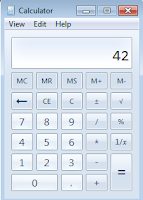## What is 7X6 ?

What is 7X6 ?7x1 = 7
7x2 = 14
7x3 = 21
7x4 = 28
7x5 = 35
7x6 = 42
7x7 = 49
7x8 = 56
7x9 = 63
7x10 = 70

This is little example, if you hard calculate you can use calculator in your smartphone. Thanks

### 2 komentarBalasBalas

Hz? Da`s een beeeeeeetje raar